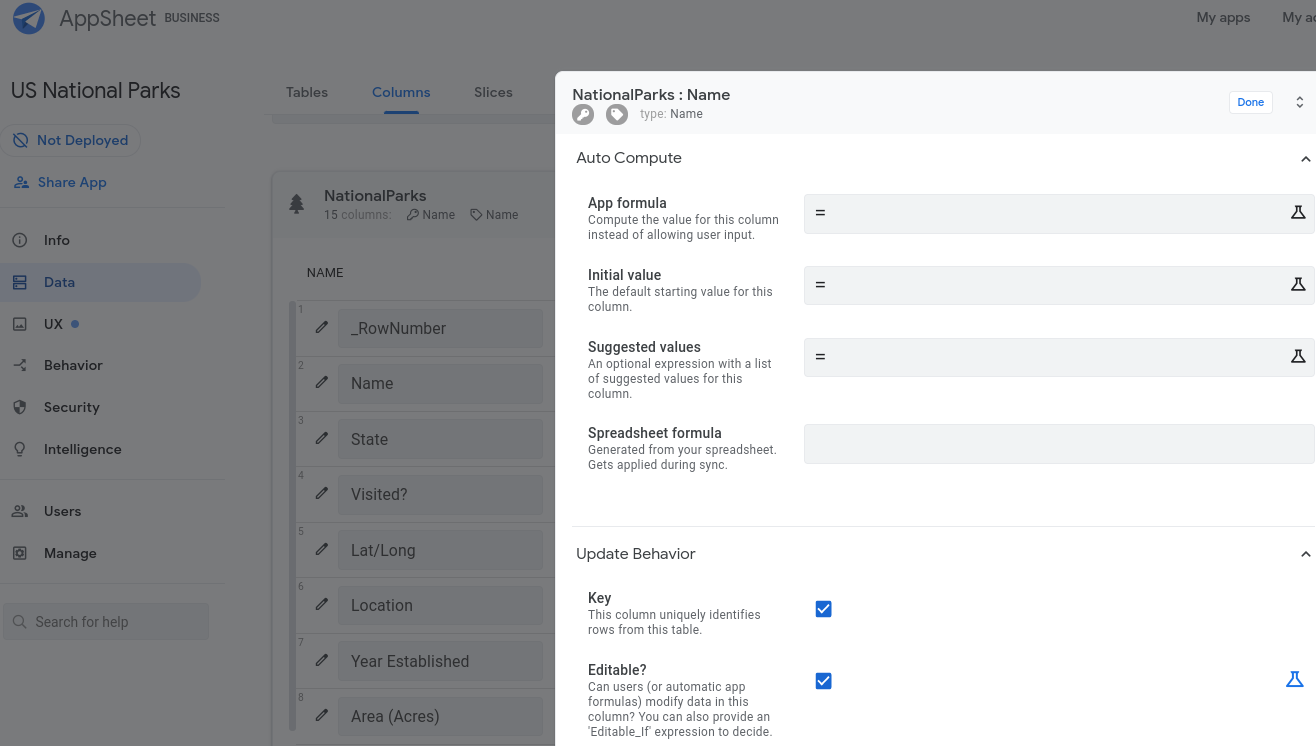# Define App formulas and Initial values

## About App formulas and Initial values

Sometimes you want calculations to run in the app while the user is interacting with the data in a form. To help you achieve that goal, you can specify an App formula for specific columns. An App formula is an expression that AppSheet evaluates whenever a user changes a value in a form (while creating a new record or editing an existing record).

Each time such a change occurs in the form, the App Formula of every affected column in the record is evaluated and the column is assigned the result of the formula evaluation. For example, if a record has a `Price` column and a `Tax` column, the `Tax` column might have an App Formula `[Price]*0.05` to compute a 5% tax automatically.

You can also specify an Initial value for every column. An Initial value expression assigns an initial or default values for a column in a new entry. If the expression is not a constant, it may be a formula that depends on other columns in the new entry. Its value will be recomputed as the new entry is filled in until the user explicitly sets the value of the column. At that point, a value has been assigned and the Initial value definition is no longer relevant for this entry.

In other words, an Initial value expression acts just like an App formula, but it has two differences: (a) the column is still editable by the user, and (b) once edited, the column is no longer automatically updated with continued re-computation of the expression.

### App formulas for virtual columns

App formulas are also used to define virtual columns. These columns do not actually exist in the spreadsheet but are only computed within the context of the app itself.

### App formulas versus spreadsheet formulas

App formulas are distinct from spreadsheet formulas. The two mechanisms are complementary and both add value to your app. Your spreadsheet is a very powerful tool to run calculations. Those calculations run only when data syncs back to the spreadsheet.

App formulas are commonly used to calculate the value of a column when the user makes updates to the app. When the changes are synced back to the spreadsheet, the computed value is saved to the corresponding spreadsheet cell.

## View and edit App formulas and Initial values

To view and edit an App formula and Initial value:

1. Open the app in the editor.
2. Go to Data > Columns
3. Expand the table you want to view or edit.
4. Click the Edit (pencil) icon to view the full set of properties for a specific column.
5. Expand the Auto Compute section.
6. Add an App formula and Initial value
7. Click Done.
8. Save the app

The formula itself is any valid AppSheet expression that matches the type of the column. For more information about the different kinds of expressions supported by AppSheet, see Expressions: The Essentials.